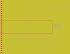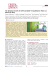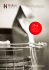# Multilinear Independent Components Analysis

## Transcription

Multilinear Independent Components Analysis
```To appear in the Proceedings of the 2005 IEEE Computer Vision and Pattern Recognition Conference (CVPR 2005), San Diego, CA, June 2005.
Multilinear Independent Components Analysis
M. Alex O. Vasilescu1,2 and Demetri Terzopoulos2,1
1
2
Department of Computer Science, University of Toronto, Toronto ON M5S 3G4, Canada
Courant Institute of Mathematical Sciences, New York University, New York, NY 10003, USA
Abstract
pertaining to scene structure, illumination, and imaging.
In this paper, we introduce a nonlinear, multifactor
model of image ensembles that generalizes conventional
ICA.1 Whereas ICA employs linear (matrix) algebra, our
Multilinear ICA (MICA) model exploits multilinear (tensor) algebra. Unlike its conventional, linear counterpart,
MICA is able to learn the interactions of multiple factors inherent to image formation and separately encode the higherorder statistics of each of these factors. Unlike our recently
proposed multilinear generalization of Eigenfaces dubbed
TensorFaces  which encodes only second order statistics associated with the different factors inherent to image
formation, MICA encodes higher order dependencies associated with the different factors.
ICA has been employed in face recognition [1, 2] and,
like PCA, it works best when person identity is the only
factor that is permitted to vary. If additional factors, such
as illumination, viewpoint, and expression can modify facial images, recognition rates decrease dramatically. This
problem is addressed by our multilinear framework. In the
context of recognition, our second contribution in this paper is a novel, multilinear projection algorithm. This algorithm projects an unlabeled test image into the multiple
factor representation spaces in order to simultaneously infer
the person, viewpoint, illumination, expression, etc., labels
associated with the test image. Equipped with this new algorithm, we demonstrate the application of multilinear ICA
to the problem of face recognition under varying viewpoint
and illumination, obtaining significantly improved results.
After reviewing the mathematical foundations of our
work in the next section, we introduce our multilinear ICA
algorithm in Section 3 and develop the associated recognition algorithm in Section 4. Section 5 describes our experiments and presents results. Section 6 concludes the paper.
Independent Components Analysis (ICA) maximizes the statistical independence of the representational components of
a training image ensemble, but it cannot distinguish between the different factors, or modes, inherent to image formation, including scene structure, illumination, and imaging. We introduce a nonlinear, multifactor model that generalizes ICA. Our Multilinear ICA (MICA) model of image ensembles learns the statistically independent components of multiple factors. Whereas ICA employs linear
(matrix) algebra, MICA exploits multilinear (tensor) algebra. We furthermore introduce a multilinear projection algorithm which projects an unlabeled test image into the N
constituent mode spaces to simultaneously infer its mode
labels. In the context of facial image ensembles, where the
mode labels are person, viewpoint, illumination, expression, etc., we demonstrate that the statistical regularities
learned by MICA capture information that, in conjunction
with our multilinear projection algorithm, improves automatic face recognition.
1
Introduction
A key problem in data analysis for pattern recognition
and signal processing is finding a suitable representation.
For historical and computational reasons, linear models
that optimally encode particular statistical properties of the
data have been broadly applied. In particular, the linear,
appearance-based face recognition method known as Eigenfaces  is founded on the principal components analysis
(PCA) of facial image ensembles . PCA encodes pairwise relationships between pixels—the second-order, correlational structure of the training image ensemble—but it ignores all higher-order pixel relationships—the higher-order
statistical dependencies. By contrast, a generalization of
PCA known as independent components analysis (ICA) 
learns a set of statistically independent components by analyzing the higher-order dependencies in the training data
in addition to the correlations. However, ICA cannot distinguish between higher-order statistics that rise from different factors, or modes, inherent to image formation—factors
2
Mathematical Background
In this section, we review the mathematics of PCA, multilinear PCA, and ICA.
1 An initial description of this work appeared as an extended abstract in
the Learning 2004 Workshop, Snowbird, UT, April, 2004.
1
People
Views
Illuminations
(a)
(b)
Figure 1: Eigenfaces and TensorFaces bases for an ensemble of 2,700 facial images spanning 75 people, each imaged under 6 viewing and
6 illumination conditions (see Section 5). (a) PCA eigenvectors (eigenfaces), which are the principal axes of variation across all images.
(b) A partial visualization of the 75 × 6 × 6 × 8560 TensorFaces representation of D, obtained as T = Z ×4 Upixels .
2.1
PCA
2.2
The principal components analysis of an ensemble of I2 images is computed by performing an SVD on a I1 × I2 data
matrix D whose columns are the “vectored” I1 -pixel “centered” images.2 The matrix D ∈ IRI1 ×I2 is a two-mode
mathematical object that has two associated vector spaces,
a row space and a column space. In a factor analysis of D,
the SVD orthogonalizes these two spaces and decomposes
the matrix as
D = UΣVT ,
Multilinear PCA
The analysis of an ensemble of images resulting from the
confluence of multiple factors, or modes, related to scene
structure, illumination, and viewpoint is a problem in multilinear algebra . Within this mathematical framework,
the image ensemble is represented as a higher-order tensor. This image data tensor D must be decomposed in order to separate and parsimoniously represent the constituent
factors. To this end, we prescribe the N -mode SVD algorithm [11, 10], a multilinear extension of the aforementioned conventional matrix SVD.
Appendix A overviews the mathematics of multilinear
analysis. Briefly, an order N > 2 tensor or N -way array D is an N -dimensional matrix comprising N spaces.
N -mode SVD is a “generalization” of conventional matrix
(i.e., 2-mode) SVD. It orthogonalizes these N spaces and
decomposes the tensor as the mode-n product, denoted ×n
(see (25)), of N -orthogonal spaces, as follows:
(1)
the product of an orthogonal column-space represented by
the left matrix U ∈ IRI1 ×J1 , a diagonal singular value matrix Σ ∈ IRJ1 ×J2 with diagonal entries σ1 ≥ σ2 ≥ . . . ≥
σp ≥ 0 called the singular values of D, and an orthogonal
row space represented by the right matrix V ∈ IRI2 ×J2 .
The eigenvectors U are also called the principal component
(or Karhunen-Loeve) directions of D (Fig. 1(a)).
Optimal dimensionality reduction in matrix principal
components analysis is obtained by truncation of the singular value decomposition (i.e., deleting eigenvectors associated with the smallest singular values).
D = Z ×1 U1 ×2 U2 . . . ×n Un . . . ×N UN .
(2)
Tensor Z, known as the core tensor, is analogous to the
diagonal singular value matrix in conventional matrix SVD
(although it does not have a simple, diagonal structure). The
core tensor governs the interaction between the mode matrices U1 , . . . , UN . Mode matrix Un contains the orthonor-
2 Each vectored-centered image is obtained by subtracting the mean image of the ensemble from each input image and identically arranging the
resulting pixels into a column vector.
2
mal vectors spanning the column space of matrix D(n) resulting from the mode-n flattening of D (see Appendix A).3
Our N -mode SVD algorithm for decomposing D according to equation (2) is:
where every column of D is a different image, W is an invertible transformation matrix that is computed by the ICA
algorithm, C = UWT are the independent components
(Fig. 2(a)), and K = W−T ΣVT are the coefficients. Various objective functions, such as those based on mutual
information, negentropy, higher-order cumulants, etc., are
presented in the literature for computing the independent
components along with different optimization methods for
extremizing these objective functions . Dimensionality
reduction with ICA is usually performed in the PCA preprocessing stage.
Alternatively, in Architecture II, ICA is applied to D
and it rotates the principal components directions such that
the coefficients are statistically independent, as follows:
1. For n = 1, . . . , N , compute matrix Un in (2) by computing the SVD of the flattened matrix D(n) and setting Un to be the left matrix of the SVD. 4
2. Solve for the core tensor as follows:
Z = D ×1 UT1 ×2 UT2 . . . ×n UTn . . . ×N UTN . (3)
Dimensionality reduction in the linear case does not have
a trivial multilinear counterpart. According to [6, 4], a
useful generalization to tensors involves an optimal rank(R1 , R2 , . . . , RN ) approximation which iteratively optimizes each of the modes of the given tensor, where each
optimization step involves a best reduced-rank approximation of a positive semi-definite symmetric matrix. This technique is a higher-order extension of the orthogonal iteration
for matrices.
The tensor basis associated with multilinear PCA is displayed in Fig. 1(b).
2.3
D = UΣVT
= UW−1 WΣVT
= CK.
where C = UW−1 is the basis matrix and K = WΣVT
are the statistically independent coefficients.
Note, that C, K and W are computed differently in the
two different architectures. Architecture I yields statistically independent bases, while Architecture II yields a “factorial code”.
Like PCA, ICA is a linear analysis method, hence it is
not well suited to the representation of multi-factor image
ensembles. To address this shortcoming, we next propose a
multilinear generalization of ICA.
ICA
The independent components analysis of multivariate data
looks for a sequence of projections such that the projected
data look as far from Gaussian as possible. ICA can be applied in two ways [1, 2]: Architecture I applies ICA to DT ,
each of whose rows is a different image, and finds a spatially independent basis set that reflects the local properties
of faces. On the other hand, Architecture II applies ICA
to D and finds a set of coefficients that are statistically independent while the basis reflects the global properties of
faces.
Architecture I: ICA starts essentially from the factor
analysis or PCA solution (1), and computes a rotation of the
principal components such that they become independent
components ; that is ICA rotates the principal component
directions U in (1) as follows:
DT
= VΣUT
= VΣW−1 WUT
T
T
= K C ,
(7)
(8)
(9)
3
Multilinear ICA
Analogously to (2), multilinear ICA is obtained by decomposing the data tensor D as the mode-n product of N mode
matrices Cn and a core tensor S, as follows:
D = S ×1 C1 ×2 C2 . . . ×n Cn . . . ×N CN .
(10)
The N -mode ICA algorithm is as follows:
1. For n = 1, . . . , N , compute the mode matrix Cn in
(10) in an architecture-dependent way (see below).
(4)
(5)
2. Solve for the core tensor as follows:
(6)
−1
−1
−1
S = D×1 C−1
1 ×2 C2 . . .×n Cn . . .×N CN . (11)
that the conventional SVD in (1) can be rewritten as D = Σ ×1
U ×2 V using mode-n products.
4 When D
(n) is a non-square matrix, the computation of Un in the
T can be persingular value decomposition (SVD) D(n) = Un ΣVn
formed efficiently, depending on which dimension of D(n) is smaller,
by decomposing either D(n) DT
= Un Σ2 UT
n and then computing
(n)
3 Note
As in ICA, there are two strategies for multilinear independent components analysis (MICA). Architecture I results in a factorial code, where each set of coefficients that
encodes people, viewpoints, illuminations, etc., is statistically independent, while Architecture II finds a set of independent bases across people, viewpoints, illuminations, etc.
T = Σ+ UT D
T
2 T
Vn
n (n) or by decomposing D(n) D(n) = Vn Σ Vn and
then computing Un = D(n) Vn Σ+ .
3
People
Views
Illuminations
(a)
(b)
Figure 2: ICA and MICA bases for an ensemble of 2,700 facial images spanning 75 people, each imaged under 6 viewing and 6 illumination conditions (see Section 5). (a) Independent components Cpixels . (b) A partial visualization of the 75 × 6 × 6 × 8560 MICA
representation of D, obtained as B = S ×4 Cpixels .
= Un Wn−1 Wn Σn VnT
= Cn Kn ,
Architecture I: Transposing the flattened data tensor D
in the nth mode and computing the ICA as in (4)–(6), we
obtain:
DT(n)
= Vn ΣTn UTn
= Vn ΣTn Wn−1 Wn UTn
(13)
= KTn CTn ,
(14)
where the mode matrices are given by
(12)
Cn = Un Wn−1 .
(15)
The columns associated with each of the mode matrices, Cn
are statistically independent. We can derive the relationship
between N -mode ICA and N -mode SVD (2) in the context
of Architecture I as follows:
D
D
= Z ×1 U1 . . . ×N UN
−T
T
= Z ×1 U1 W1T W1−T . . . ×N UN WN
WN
−T
= Z ×1 C1 W1−T . . . ×N CN WN
4
−T
.
where the core tensor S = Z ×1 W1−T . . . ×N WN
Architecture II: Flattening the data tensor D in the nth
mode and computing the ICA as in (7)–(9), we obtain:
= Un Σn VnT
=
=
=
=
=
Z ×1 U1 . . . ×N UN
−1
Z ×1 U1 W1−1 W1 . . . ×N UN WN
WN
Z ×1 C1 W1 . . . ×N CN WN
(Z ×1 W1 . . . ×N WN ) ×1 C1 . . . ×N CN
S ×1 C1 . . . ×N CN ,
where the core tensor S = Z ×1 W1 . . . ×N WN .
−T
= (Z ×1 W1−T . . . ×N WN
) ×1 C1 . . . ×N CN
= S ×1 C1 . . . ×N CN ,
D(n)
(19)
Architecture II results in a set of basis vectors that are statistically independent across the different modes. Note that
the Wn in (19) differ from those in (15).
We can derive the relationship between N -mode ICA
and N -mode SVD (2) in the context of Architecture II as
follows:
where the mode matrices are given by
Cn = Un WnT .
(17)
(18)
Recognition
Our approach performs a multilinear ICA decomposition of
the tensor D of vectored training images dp,v,l ,
D = B ×1 Cpeople ×2 Cviews ×3 Cillums ,
(16)
4
(20)
cv
cl
l4
l3
=
[l
l2
1
..
[ v1
]
.
v2
p 1 v1 l 4
p 1 v1 l 3
p1v3l4
p 1 v2 l 3
p1v1l2
p 1 v2 l 2
v4 ...
v3
p1v2l4
]
cl
p1v4l4
p 1 v3 l 3
p 1 v3 l 2
p1v4l3
p 1 v4 l 2
l4
l3
P
Views
x4
=
[
l2
l1
..
.
[ v1
]
v2
p1v1l4
p1v2l4
p1v1l3
p1v1l2
p1v3l4
p1v2l3
p1v2l2
v4 ...
v3
p1v4l4
p 1 v 3l 3
p 1 v 3l 2
p1v4l3
p 1 v4 l 2
p1
p 1 v1 l 1
p 1 v2 l 1
p1v3l1
p1v4l1
p1
p1v1l1
p1v2l1
p 1 v 3l 1
p1v4l1
p2
p 2 v1 l 1
p 2 v2 l 1
p2v3l1
p2v4l1
p2
p2v1l1
p2v2l1
p 2 v 3l 1
p2v4l1
p 3 v1 l 1
p 3 v2 l 1
p3v3l1
p3v4l1
p3
p3v1l1
p3v2l1
p 3 v 3l 1
p3v4l1
p 4 v1 l 1
p 4 v2 l 1
p4v3l1
p4v4l1
p4
p4v1l1
p4v2l1
p 4 v 3l 1
p 4v4 l 1
p 5 v1 l 1
p 5 v2 l 1
p5v3l1
p5v4l1
p5
p5v1l1
p5v2l1
p 5 v 3l 1
p 5v4 l 1
p3
p4
p5
]
Views
[
[
cp
cv
Illuminations
Illuminations
cp
... ]
... ]
People
People
(a)
(b)
Figure 3: (a) Image representation dT = B ×1 cTp ×2 cTv ×3 cTl . (b) Given an unlabeled test image dT , the associated coefficient vectors
cp , cv , cl are computed by decomposing the response tensor R = P ×4 dT using the N -mode SVD algorithm.
extracting a set of mode matrices—the matrix Cpeople containing row vectors cTp of coefficients for each person p,
the matrix Cviews containing row vectors cTv of coefficients
for each view direction v, and the matrix Cillums containing
row vectors cTl of coefficients for each illumination direction l—and a MICA basis tensor
sor framework and the statistical independence properties
of ICA.
From (20), MICA represents the unlabeled, test image
by a set of coefficient vectors:
B = S ×4 Cpixels
where the coefficient vector cp encodes the person, the coefficient vector cv encodes the viewpoint, and the coefficient
vector cl encodes the illumination. The multilinear representation of an image is illustrated in Fig. 3(a).
The response tensor, illustrated in Fig. 3(b), is computed
as
R = P ×4 dT ,
(24)
dT = B ×1 cTp ×2 cTv ×3 cTl ,
(21)
that governs the interaction between the different mode matrices (Fig. 2(b)). Given an unlabeled test image, recognition is performed by inferring the associated coefficient
vectors.
The recognition algorithm for TensorFaces proposed in
our earlier work  was based on a linear projection approach.5 It computed a set of linear projection operators for
each mode, which yielded a set of candidate coefficients per
mode.
We will now develop a multilinear method for simultaneously inferring the identity, illumination, viewpoint, etc.,
coefficient vectors of an unlabeled, test image. Our method
maps an image from the pixel space to N different constituent mode spaces. We obtain a new recognition algorithm that is based on the multilinear structure of the ten-
(23)
(22)
where the “projection tensor” P is obtained by retensorizing matrix P(pixels) = B−T
(pixels) (the matrix B(pixels) is the
pixel-mode flattening of tensor B).
The response R has the structure (cp ◦ cv ◦ cl ).6 It is a
tensor of rank (1,1,1) as it is the outer product of the three
coefficient vectors cp , cv , and cl .
The structure of R enables us to compute the three coefficient vectors via a tensor decomposition using the N mode SVD algorithm. This is because the fibers (columns,
rows, tubes) of R are multiples of their corresponding coefficient vectors (cp , cv , cl ) (cf. the boxed rows/columns
in Fig. 3(b)). Thus, flattening R in each mode yields rank
1 matrices, enabling the modal SVD to compute the corresponding coefficient vector. The N -mode SVD thus maps
We obtain the vector of “person coefficients” p associated with d as
T
follows: cT
p = P ×2 d , where the linear projection operator P =
Upixels Σ−1 .
6 We can show that P × dT = I × (cT ⊗ cT ⊗ cT ), where ⊗
4
4
v
p
l
denotes the Kronecker product and I is the re-tensorized identity matrix
I(pixels) = (B+(pixels) B(pixels) )T .
5 In the PCA (eigenfaces) technique, one decomposes a data matrix D
of known, training facial images dd into a basis matrix Upixels and a matrix
of coefficient vectors. In our tensor notation, an unlabeled, test facial image
d can be decomposed and represented as
dT = Σ ×1 cT
p ×2 Upixels .
5
the response tensor R into N different mode spaces that explicitly account for the contribution of each mode—person,
viewpoint, illumination.
In particular, note that the person coefficient vector cp
is the left singular matrix of the SVD of R(people) . To recognize the person in the test image dT , we apply a normalized nearest neighbor classification scheme by computing
normalized scalar products between cp and each of the row
vectors of the people mode matrix Cpeople .
5
applied our multilinear ICA (MICA) algorithm to a multimodal face recognition problem involving multiple people imaged under different viewpoints and illuminations.
We also introduced a multilinear projection algorithm for
recognition, which projects an unlabeled test image into
the N constituent mode spaces to infer its mode labels—
person, viewpoint, illumination, etc. In our experiments, we
obtained improved recognition results relative to the prior
methods under consideration, because MICA disentangles
the multiple factors inherent to image formation and explicitly represents the higher-order statistics associated with
each factor.
Experiments
In our experiments, we employ gray-level facial images of
75 subjects. Each subject is imaged from 15 different viewpoints (θ = −35◦ to +35◦ in 5◦ steps on the horizontal
plane φ = 0◦ ) under 15 different illuminations (θ = −35◦
to +35◦ in 5◦ steps on an inclined plane φ = 45◦ ). Fig. 4(b)
shows the full set of 225 images for one of the subjects
with viewpoints arrayed horizontally and illuminations arrayed vertically. The image set was rendered from a 3D
scan of the subject shown boxed in Fig. 4(a). The 75 scans
shown in the figure were recorded using a CyberwareT M
3030PS laser scanner and are part of the 3D morphable
faces database created at the University of Freiburg .
Recognition Experiment
Training: 75 people, 6 viewpoints (θ =
±35, ±20, ±5, φ = 0), 6 illuminations (θ = 45, φ = 90 + δ,
δ = ±35, ±20, ±5)
Testing: 75 people, 9 viewpoints (φ =
0 ± 10, ±15, ±25, ± = 30), 9
illuminations (θ = 90 + δ, δ =
±35, ±20, ±5, θ = 0)
PCA
ICA
MPCA
MICA
83.9%
89.5%
93.4%
98.14%
Acknowledgements
This research was funded by the Technical Support Working Group (TSWG) of the US Department of Defense. The
3D Morphable faces database was obtained courtesy of
Prof. Sudeep Sarkar of the University of South Florida as
part of the USF HumanID 3D database.
A
A tensor is a higher order generalization of a vector (first
order tensor) and a matrix (second order tensor). Tensors are multilinear mappings over a set of vector spaces.
The order of tensor A ∈ IRI1 ×I2 ×...×IN is N . Elements of A are denoted as Ai1 ...in ...iN or ai1 ...in ...iN , where
1 ≤ in ≤ In . In tensor terminology, matrix column
vectors are referred to as mode-1 vectors and row vectors
as mode-2 vectors. The mode-n vectors of an Nth order tensor A are the In -dimensional vectors obtained from
A by varying index in while keeping the other indices
fixed. The mode-n vectors are the column vectors of matrix
A(n) ∈ IRIn ×(I1 I2 ...In−1 In+1 ...IN ) that results by mode-n
flattening the tensor A (see Fig. 1 in ). The n-rank of
A ∈ IRI1 ×I2 ×...×IN , denoted Rn , is defined as the dimension of the vector space generated by the mode-n vectors.
A generalization of the product of two matrices is the
product of a tensor and a matrix. The mode-n product
of a tensor A ∈ IRI1 ×I2 ×...×In ×...×IN by a matrix M ∈
IRJn ×In , denoted by A ×n M, is the I1 × . . . × In−1 ×
Jn × In+1 × . . . × IN tensor
As the above table shows, in our experiments with
16, 875 images captured from the University of Freiberg 3D
Morphable Faces Database, MICA yields better recognition
rates than PCA (eigenfaces) and ICA in scenarios involving the recognition of people imaged in previously unseen
viewpoints and illuminations. MICA training employed an
ensemble of 2,700 images. Fig. 2(b) illustrates the MICA
basis derived from the training ensemble, while Fig. 2(a)
illustrates the ICA basis.
6
Multilinear Math
(A ×n M)i1 ...in−1 jn in+1 ...iN =
ai1 ...in−1 in in+1 ...iN mjn in .
Conclusion
Motivated by the reported outperformance in the face recognition literature of PCA by ICA in the linear case where
only a single factor is allowed to vary, and the outperformance of PCA by TensorFaces when multiple factors are
allowed to vary, it is natural to ask whether there a multilinear generalization of ICA and if its performance is better than the other two methods. In this paper, we developed a multilinear generalization of ICA and successfully
(25)
in
The mode-n product can be expressed in terms of flattened
matrices as B(n) = MA(n) . The mode-n product of a tensor and a matrix is a special case of the inner product in
multilinear algebra and tensor analysis. Note that for tensors and matrices of the appropriate sizes, A ×m U ×n V =
A ×n V ×m U and (A ×n U) ×n V = A ×n (VU).
6
-35
-30
-25
-20
-15
-10
-5
0
5
10
15
20
25
30
35
-35
-30
-25
-20
-15
-10
-5
0
5
10
15
20
25
30
35
(a)
(b)
Figure 4: (a) 3D scans of 75 subjects, recorded using a CyberwareT M 3030PS laser scanner as part of the University of Freiburg 3D
morphable faces database . (b) Full set of facial images for a subject (boxed head in (a)), viewed from 15 different viewpoints (across)
under 15 different illuminations (down). The dashed-boxed images served as training images; the solid-boxed images served as testing
images.
References

 M.S. Bartlett. Face Image Analysis by Unsupervised Learning. Kluwer Academic, Boston, 2001.
 M.S. Bartlett, J.R. Movellan, and T.J. Sejnowski. Face recognition by independent component analysis. IEEE Transactions on Neural Networks, 13(6):1450–1464, 2002.
 V. Blanz and T.A. Vetter. Morphable model for the synthesis of 3d faces. In SIGGRAPH 99 Conference Proceedings,
pages 187–194. ACM SIGGRAPH, 1999.
 L. de Lathauwer, B. de Moor, and J. Vandewalle. On the best
rank-1 and rank-(R1 , R2 , . . . , Rn ) approximation of higherorder tensors. SIAM Journal of Matrix Analysis and Applications, 21(4):1324–1342, 2000.
 A. Hyvärinen, J. Karhunen, and E. Oja. Independent Component Analysis. Wiley, New York, 2001.
 P. M. Kroonenberg and J. de Leeuw. Principal component
analysis of three-mode data by means of alternating least
squares algorithms. Psychometrika, 45:69–97, 1980.
 L. Sirovich and M. Kirby. Low dimensional procedure for



7
the characterization of human faces. Journal of the Optical
Society of America A., 4:519–524, 1987.
J. Friedman T. Hastie, R. Tibshirani. The Elements of Statistical Learning: Data Mining, Inference, and Prediction.
Springer, New York, 2001.
M. A. Turk and A. P. Pentland. Face recognition using eigenfaces. In Proceedings IEEE Computer Society Conference on
Computer Vision and Pattern Recognition, pages 586–590,
Hawai, 1991.
M.A.O. Vasilescu and D. Terzopoulos. Multilinear analysis
for facial image recognition. In Proc. Int. Conf. on Pattern
Recognition, volume 2, pages 511–514, Quebec City, August
2002.
M.A.O. Vasilescu and D. Terzopoulos. Multilinear analysis
of image ensembles: Tensorfaces. In Proc. European Conf.
on Computer Vision (ECCV 2002), pages 447–460, Copenhagen, Denmark, May 2002.
```

### [email protected] [email protected]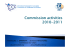### Cranial Nerves Atlas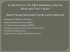### U libre de Bruxelle - International Communication Association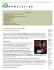### Ica news - Wilson Elser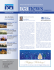### Innovative data processing methods for gradient airborne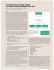### FYE September 30, 2009 - Interstitial Cystitis Association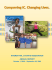### SVD++ and PMF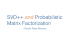### Denoising and Completion of 3D Data via Multidimensional### toournation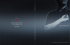### Illustrators and Animators at the Top of Their Fields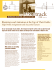### A Robust Multilinear Model Learning Framework for 3D Faces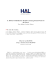### View - GFZ publication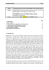### FFR : Clinical studies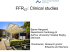### Tensor Decompositions and Applications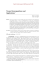### November 2010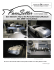### New Media and Internet Communication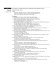### PROFILE PROJECTORS PJ-H30 Series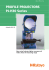### December 2010 - Kentucky Porsche Club of America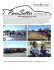### Classical Electrodynamics - Duke Physics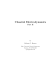### the Annual Report - Maryland Institute College of Art